Ask your WordPress questions! Pay money and get answers fast!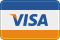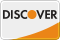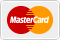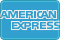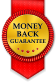# Featured Image Fallback and remove first image in post. WordPress

• SOLVED

I am having issues with my code for featured posts. Here what it does:

1. If has featured image > Show
2. If doesnt > Show first image in post (also need to delete first image in post)
3. If no image in post and no featured image > default.jpg

What i need is to <strong>delete the first image in the post</strong> so it does not repeat (#2). Any ideas?

Here is function:
``` function get_fbimage() { if ((function_exists('has_post_thumbnail')) && (has_post_thumbnail())) { \$src = wp_get_attachment_image_src( get_post_thumbnail_id(\$post->ID), '', '' ); \$fbimage = \$src; } else { global \$post, \$posts; \$fbimage = ''; \$output = preg_match_all('/<img.+src=[\'"]([^\'"]+)[\'"].*>/i', \$post->post_content, \$matches); \$fbimage = \$matches  ; } if(empty(\$fbimage)) { \$fbimage = "http://www.example.com/defualt.jpg"; } return \$fbimage; } ```

Here is the php
``` <?php if ( function_exists( 'has_post_thumbnail') && (has_post_thumbnail()) ) : echo get_the_post_thumbnail(\$post->ID); else :?> <img src="<?php echo get_fbimage();?>" /> <?php endif;?> ```

2013-07-02

try this

```<?php function get_fbimage() { if ((function_exists('has_post_thumbnail')) && (has_post_thumbnail())) { \$src = wp_get_attachment_image_src( get_post_thumbnail_id(\$post->ID), '', '' ); \$fbimage = \$src; } else { global \$post, \$posts; add_filter('the_content', 'remove_first_image'); \$fbimage = ''; \$output = preg_match_all('/<img.+src=[\'"]([^\'"]+)[\'"].*>/i', \$post->post_content, \$matches); \$fbimage = \$matches  ; } if(empty(\$fbimage)) { \$fbimage = "http://www.example.com/defualt.jpg"; } return \$fbimage; } function remove_first_image (\$content) { \$content = preg_replace("/<img[^>]+\>/i", "", \$content, 1); return \$content; } ```

can you share your code how you are calling content of the post to display?

so basically this function will remove the first image , and you can call it via filter

```function remove_first_image (\$content) { \$content = preg_replace("/<img[^>]+\>/i", "", \$content, 1); return \$content; } add_filter('the_content', 'remove_first_image'); ```

This actually works with out the
``` add_filter('the_content', 'remove_first_image');```

Because with that all pages loose the first image (that are not blog post with a featured image).

2013-07-01

``` function catch_that_image() { global \$post, \$posts; \$first_img = ''; ob_start(); ob_end_clean(); \$output = preg_match_all('/<img.+src=[\'"]([^\'"]+)[\'"].*>/i', \$post->post_content, \$matches); \$first_img = \$matches; if(empty(\$first_img)) { \$first_img = "/path/to/default.png"; } return \$first_img; } ```
``` if ( get_the_post_thumbnail(\$post_id) != '' ) { echo '<a href="'; the_permalink(); echo '" class="thumbnail-wrapper">'; the_post_thumbnail(); echo '</a>'; } else { echo '<a href="'; the_permalink(); echo '" class="thumbnail-wrapper">'; echo '<img src="'; echo catch_that_image(); echo '" alt="" />'; echo '</a>'; } ```